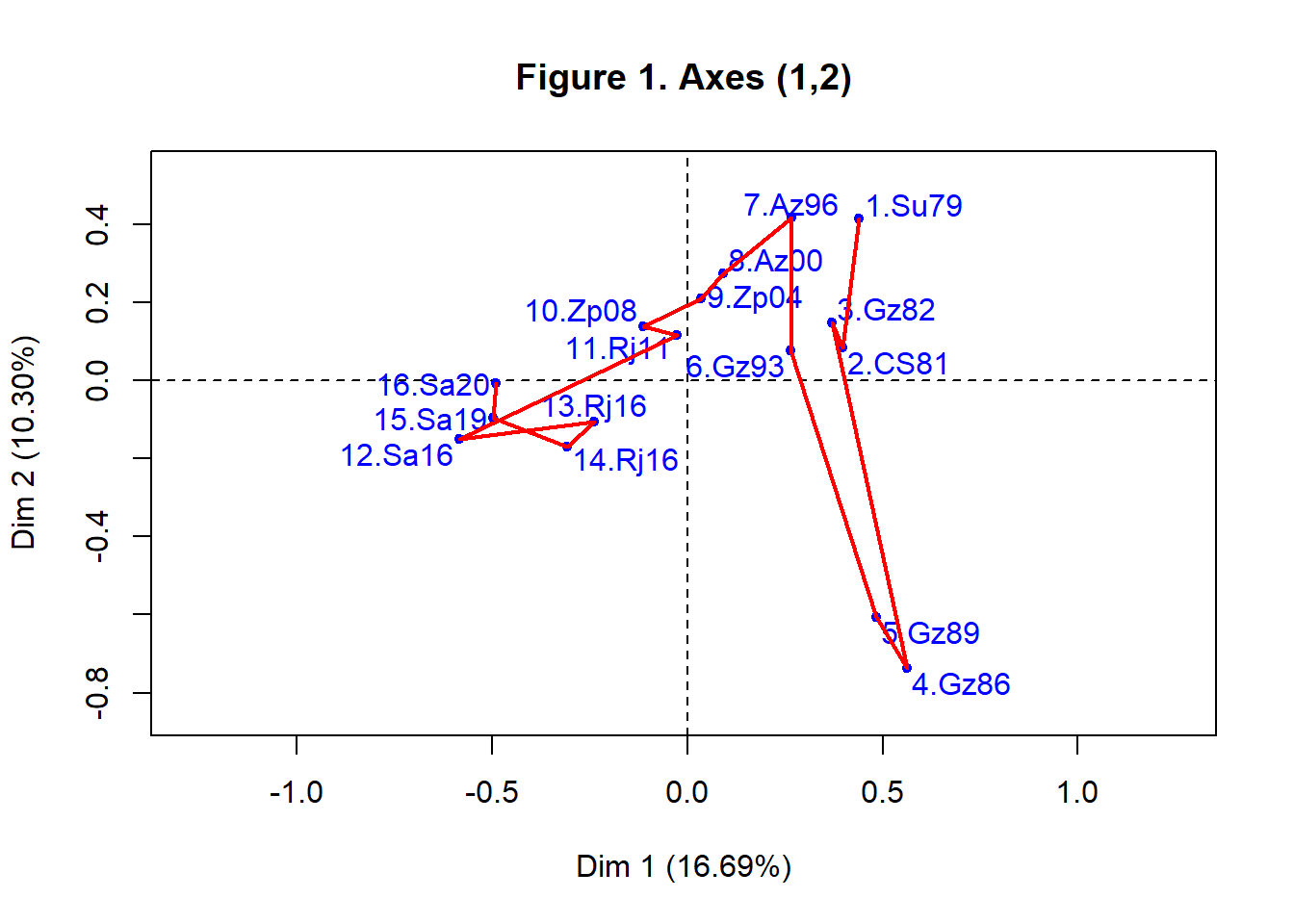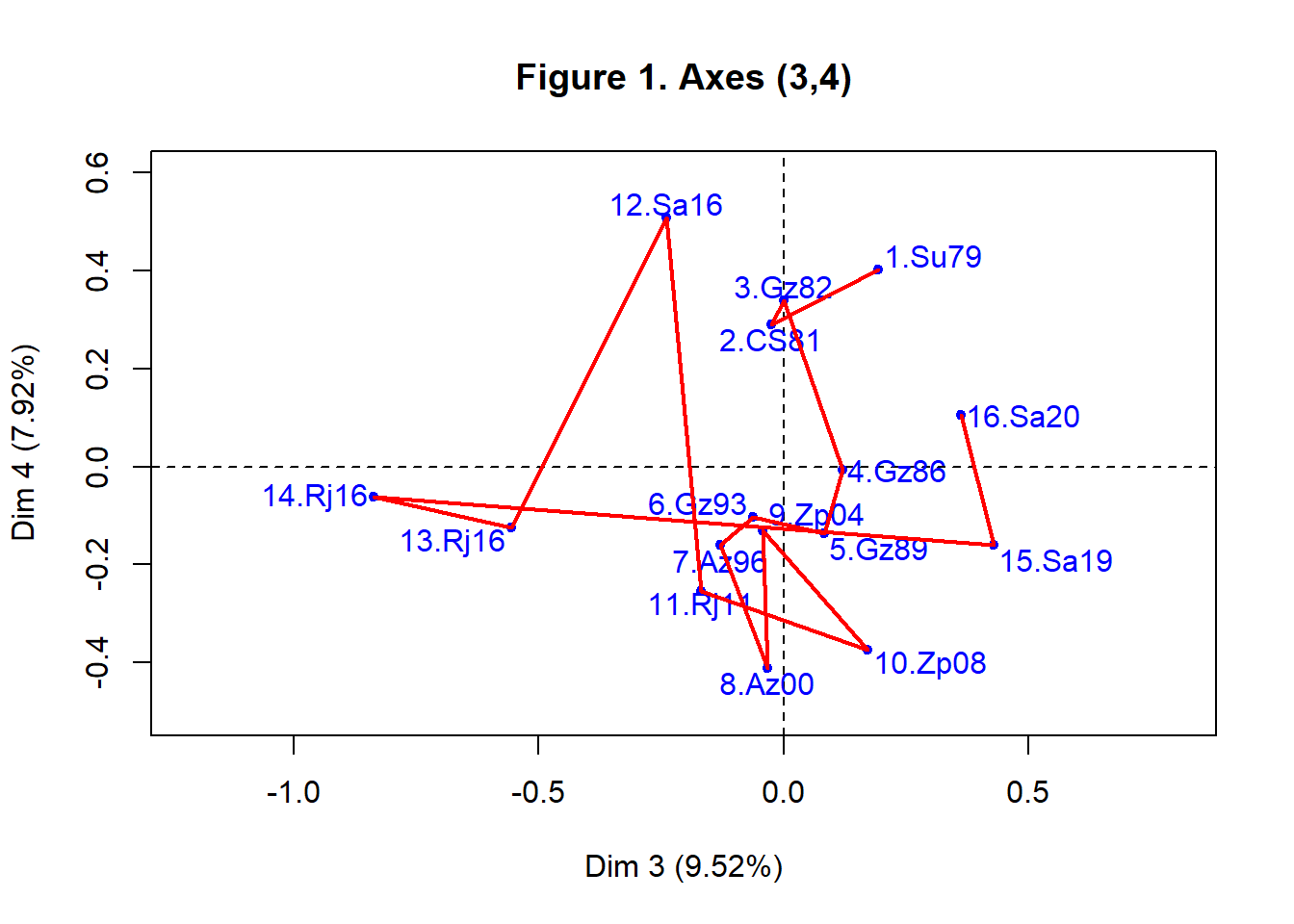# JADT 2022. 4.2. Correspondence analysis

### 4.2. Correspondence analysis

After performing CA, 5 axes associated to the above-average eigenvalues (equal to 0.153, 0.094, 0.087, 0.073 and 0.064, respectively) have been retained. All together, they keep 51.4% of the total variance (respectively, 16.7; 10.3; 9.5; 7.9; 7.0). The chronology is indicated here by the rank of the speeches in the series (from 1 to 16), not the year.

###### `mean(resCA\$eig[,1])`

Figure 1 represents the speeches on the first two CA planes, (1,2) and (3,4). In this case, the temporal changes unfold along the first
three axes.

The second axis shows how the first four speeches evolve, while the first axis describes the changes from 4.Gz86 to 12.Sa16, drawing a rough parabola, a usual shape when vocabulary evolves over time.

Finally, axis 3 informs about what is characterizing the last four speeches, which is the opposition between 13.Rj16 and 14. Rj16, at the negative part of this axis, and 15.Sa19 and 16.Sa20, on the positive part. It can be assumed that it is an ideological opposition between the candidates of two opposing parties. They are competing for the head of government by means of motions of censure and election repetitions. As our aim is to present the method and not to analyse these speeches in detail, we do not say more here. Let us just add that both axes 4 and 5 underlines the particularity of the speech 12.Sa16 in relation to those close in time.

###### ```plot(resCA, selDoc="ALL", selWord=NULL, axes=c(1,2), graph.type = c("classic"), title="Figure 1. Axes (1,2)") lines(resCA\$row\$coord[1:16,1],resCA\$row\$coord[1:16,2],lwd=2,col="red")```###### ```plot(resCA, selDoc="ALL", selWord=NULL, axes=c(3,4), graph.type = c("classic"), title="Figure 1. Axes (3,4)") lines(resCA\$row\$coord[1:16,3],resCA\$row\$coord[1:16,4],lwd=2,col="red")```